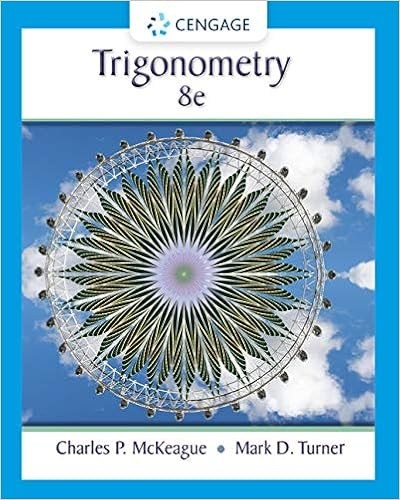# Math 1013 Tutorial 6 (Revision Exercises for Mid Term Exam)

• Test Prep
• 3

This preview shows page 1 out of 3 pages.

##### We have textbook solutions for you!
The document you are viewing contains questions related to this textbook.The document you are viewing contains questions related to this textbook.
Chapter A / Exercise 37
Trigonometry
McKeague/TurnerExpert VerifiedUnformatted text preview: Math 1013 Tutorial 6 (Revision Exercises for Mid Term Exam) (1) Page | 1 Derivative of the Inverse Function 1) Evaluation of Composite Functions If () = √ and () = − , find the compositions 2) , , . Domain of a Function Find the Domain and Range of the following Functions : (a) () = ( − )√ + (b) ) = ( − ) (c) () = (+)(−) (d) () = √ − (e) () = + Example 1 − √+ 3) State the condition (criteria) that a function () has an inverse function − (). 4) Suppose that −− () = { − ≠ = (a) Find → (). (b) Is () continuous at = ? (c) Is () differentiable at = ? Math 1013 Tutorial 6 (Revision Exercises for Mid Term Exam) 5) 6) () = − Evaluate →∞ () and →−∞ () for 8) √− (a) Find →− (). (a) (b) Find →+ (). (b) (c) What is the value of () so that () is continuous at = . Why? Evaluate the Limits : (a) → √ + () = ( − √ + ) +√ + √++ (c) → (d) →∞ ( + (e) →−∞ − + < = > Determine values of the constants and for which g is continuous at − + → =. 10) Determining the unknown constant (−)√+ −√+ √ () = { ) − ≤ > For what value of for which ′() exist . − + √ + + 11) Find →+ () (a) If () = () is definded by () = { 9) (b) (f) 7) Page | 2 () = {√ − ≤ − < < ≥ Compute the following limits or state that they do not exist. (a) →−− () (b) →−+ () (c) →− () (d) →− () (e) →+ () (f) → () = + (b) = ( + ) (c) = (( )) (d) = (√ ) (f) = (+ ) (g) = ( + + ) (h) 12) , if : () = Prove that → (i) () = and → (+)/ (−)/ (+)/ do not exist. Math 1013 Tutorial 6 (Revision Exercises for Mid Term Exam) 13) Given that () = , ′ () = , () = , ′ () = , ′ () () = , =. () 18) (a) Suppose the slope of the curve = − () at (4, 7) is . Find ′() . (a) If () = , find ′() . (b) If () = (()) , find ′(). (c) If () = ()()() , find ′() . () Page | 3 (b) Find (− )′() if () 19) () = + + . Inverse functions Find the inverse functions and the derivatives of their inverse functions for the following functions : (d) 14) If () = (() )(() ) √() , find ′(). Find the equations of tangents to the following curves at the given points : − (a) + (b) + = ; (, ) (c) ( + − ) = ( + ) ; (2, 2) (d) = ; (, ) 16) Find (a) (c) (e) 17) ( + ) ( + ) ( ) (b) (d) (b) () = + (c) () = | + | for ≥ − . + − + < () = { + ≥ where is a constant. Find such that is continuous for all . A function is defined by 21) The graph of the function f is given as follows. () = − and () = + . Find √ + = + , (1, 0) () = √ + , Find ′() and ′(). () = 20) 15) (a) ( + ) (( ) ) Find the derivates of the following functions : (a) () = − ( ) (b) () = − ( + ) (c) () = − √ (d) () = (− ) (a) →−− () (b) →−− (()) (c) →−− (()) (d) →−+ (()) 22) Let () = √|| || . Determine if ′() exists or not. Justify your answer. ...
View Full Document

•••